﻿ Orbit Maneuvers | Aerospace Engineering

## Orbit Maneuvers

### Orbit Meccanics:

At some point during the lifetime of most space vehicles or satellites, we must change one or more of the orbital elements. For example, we may need to transfer from an initial parking orbit to the final mission orbit, rendezvous with or intercept another spacecraft, or correct the orbital elements to adjust for the perturbations discussed in the previous section. Most frequently, we must change the orbit altitude, plane, or both. To change the orbit of a space vehicle, we have to change its velocity vector in magnitude or direction. Most propulsion systems operate for only a short time compared to the orbital period, thus we can treat the maneuver as an impulsive change in velocity while the position remains fixed. For this reason, any maneuver changing the orbit of a space vehicle must occur at a point where the old orbit intersects the new orbit. If the orbits do not intersect, we must use an intermediate orbit that intersects both. In this case, the total maneuver will require at least two propulsive burns.

Orbit Altitude ChangesThe most common type of in-plane maneuver changes the size and energy of an orbit, usually from a low-altitude parking orbit to a higher-altitude mission orbit such as a geosynchronous orbit. Because the initial and final orbits do not intersect, the maneuver requires a transfer orbit. Figure 4.11 represents a Hohmann transfer orbit. In this case, the transfer orbit’s ellipse is tangent to both the initial and final orbits at the transfer orbit’s perigee and apogee respectively. The orbits are tangential, so the velocity vectors are collinear, and the Hohmann transfer represents the most fuel-efficient transfer between two circular, coplanar orbits. When transferring from a smaller orbit to a larger orbit, the change in velocity is applied in the direction of motion; when transferring from a larger orbit to a smaller, the change of velocity is opposite to the direction of motion.

The total change in velocity required for the orbit transfer is the sum of the velocity changes at perigee and apogee of the transfer ellipse. Since the velocity vectors are collinear, the velocity changes are just the differences in magnitudes of the velocities in each orbit. If we know the initial and final orbits, rA and rB, we can calculate the total velocity change using the following equations:Note that equations (4.59) and (4.60) are the same as equation (4.6), and equations (4.61) and (4.62) are the same as equation (4.45).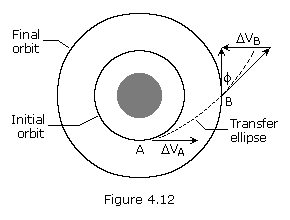Ordinarily we want to transfer a space vehicle using the smallest amount of energy, which usually leads to using a Hohmann transfer orbit. However, sometimes we may need to transfer a satellite between orbits in less time than that required to complete the Hohmann transfer. Figure 4.12 shows a faster transfer called the One-Tangent Burn. In this instance the transfer orbit is tangential to the initial orbit. It intersects the final orbit at an angle equal to the flight path angle of the transfer orbit at the point of intersection. An infinite number of transfer orbits are tangential to the initial orbit and intersect the final orbit at some angle. Thus, we may choose the transfer orbit by specifying the size of the transfer orbit, the angular change of the transfer, or the time required to complete the transfer. We can then define the transfer orbit and calculate the required velocities.

For example, we may specify the size of the transfer orbit, choosing any semi-major axis that is greater than the semi-major axis of the Hohmann transfer ellipse. Once we know the semi-major axis of the ellipse, atx, we can calculate the eccentricity, angular distance traveled in the transfer, the velocity change required for the transfer, and the time required to complete the transfer. We do this using equations (4.59) through (4.63) and (4.65) above, and the following equations: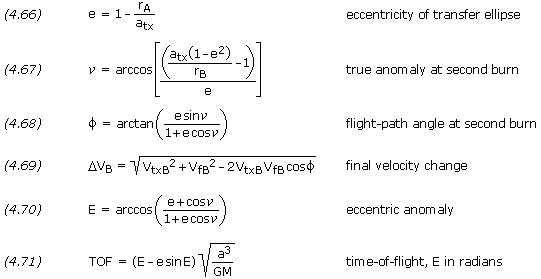Another option for changing the size of an orbit is to use electric propulsion to produce a constant low-thrust burn, which results in a spiral transfer. We can approximate the velocity change for this type of orbit transfer by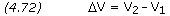where the velocities are the circular velocities of the two orbits.

Orbit Plane Changes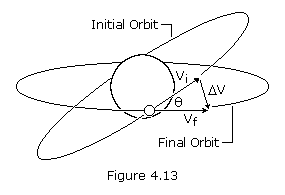To change the orientation of a satellite’s orbital plane, typically the inclination, we must change the direction of the velocity vector. This maneuver requires a component ofV to be perpendicular to the orbital plane and, therefore, perpendicular to the initial velocity vector. If the size of the orbit remains constant, the maneuver is called asimple plane change. We can find the required change in velocity by using the law of cosines. For the case in which Vf is equal to Vi, this expression reduces to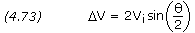where Vi is the velocity before and after the burn, andis the angle change required.

From equation (4.73) we see that if the angular change is equal to 60 degrees, the required change in velocity is equal to the current velocity. Plane changes are very expensive in terms of the required change in velocity and resulting propellant consumption. To minimize this, we should change the plane at a point where the velocity of the satellite is a minimum: at apogee for an elliptical orbit. In some cases, it may even be cheaper to boost the satellite into a higher orbit, change the orbit plane at apogee, and return the satellite to its original orbit.

Typically, orbital transfers require changes in both the size and the plane of the orbit, such as transferring from an inclined parking orbit at low altitude to a zero-inclination orbit at geosynchronous altitude. We can do this transfer in two steps: a Hohmann transfer to change the size of the orbit and a simple plane change to make the orbit equatorial. A more efficient method (less total change in velocity) would be to combine the plane change with the tangential burn at apogee of the transfer orbit. As we must change both the magnitude and direction of the velocity vector, we can find the required change in velocity using the law of cosines,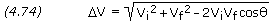where Vi is the initial velocity, Vf is the final velocity, andis the angle change required. Note that equation (4.74) is in the same form as equation (4.69).

As can be seen from equation (4.74), a small plane change can be combined with an altitude change for almost no cost inV or propellant. Consequently, in practice, geosynchronous transfer is done with a small plane change at perigee and most of the plane change at apogee.

Another option is to complete the maneuver using three burns. The first burn is a coplanar maneuver placing the satellite into a transfer orbit with an apogee much higher than the final orbit. When the satellite reaches apogee of the transfer orbit, a combined plane change maneuver is done. This places the satellite in a second transfer orbit that is coplanar with the final orbit and has a perigee altitude equal to the altitude of the final orbit. Finally, when the satellite reaches perigee of the second transfer orbit, another coplanar maneuver places the satellite into the final orbit. This three-burn maneuver may save propellant, but the propellant savings comes at the expense of the total time required to complete the maneuver.

When a plane change is used to modify inclination only, the magnitude of the angle change is simply the difference between the initial and final inclinations. In this case, the initial and final orbits share the same ascending and descending nodes. The plane change maneuver takes places when the space vehicle passes through one of these two nodes.

In some instances, however, a plane change is used to alter an orbit’s longitude of ascending node in addition to the inclination. An example might be a maneuver to correct out-of-plane errors to make the orbits of two space vehicles coplanar in preparation for a rendezvous. If the orbital elements of the initial and final orbits are known, the plane change angle is determined by the vector dot product. If ii andi are the inclination and longitude of ascending node of the initial orbit, and if andf are the inclination and longitude of ascending node of the final orbit, then the angle between the orbital planes,, is given by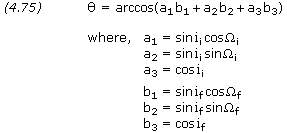The plane change maneuver takes place at one of two nodes where the initial and final orbits intersect. The latitude and longitude of these nodes are determined by the vector cross product. The position of one of the two nodes is given byKnowing the position of one node, the second node is simply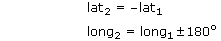Orbit Rendezvous

Orbital transfer becomes more complicated when the object is to rendezvous with or intercept another object in space: both the interceptor and the target must arrive at the rendezvous point at the same time. This precision demands a phasing orbit to accomplish the maneuver. A phasing orbit is any orbit that results in the interceptor achieving the desired geometry relative to the target to initiate a Hohmann transfer. If the initial and final orbits are circular, coplanar, and of different sizes, then the phasing orbit is simply the initial interceptor orbit. The interceptor remains in the initial orbit until the relative motion between the interceptor and target results in the desired geometry. At that point, we would inject the interceptor into a Hohmann transfer orbit.

Launch Windows

Similar to the rendezvous problem is the launch-window problem, or determining the appropriate time to launch from the surface of the Earth into the desired orbital plane. Because the orbital plane is fixed in inertial space, the launch window is the time when the launch site on the surface of the Earth rotates through the orbital plane. The time of the launch depends on the launch site’s latitude and longitude and the satellite orbit’s inclination and longitude of ascending node.

Orbit Maintenance

Once in their mission orbits, many satellites need no additional orbit adjustment. On the other hand, mission requirements may demand that we maneuver the satellite to correct the orbital elements when perturbing forces have changed them. Two particular cases of note are satellites with repeating ground tracks and geostationary satellites.

After the mission of a satellite is complete, several options exist, depending on the orbit. We may allow low-altitude orbits to decay and reenter the atmosphere or use a velocity change to speed up the process. We may also boost satellites at all altitudes into benign orbits to reduce the probability of collision with active payloads, especially at synchronous altitudes.V Budget

To an orbit designer, a space mission is a series of different orbits. For example, a satellite might be released in a low-Earth parking orbit, transferred to some mission orbit, go through a series of resphasings or alternate mission orbits, and then move to some final orbit at the end of its useful life. Each of these orbit changes requires energy. TheV budget is traditionally used to account for this energy. It sums all the velocity changes required throughout the space mission life. In a broad sense theV budget represents the cost for each mission orbit scenario.

The Hyperbolic Orbit

The discussion thus far has focused on the elliptical orbit, which will result whenever a spacecraft has insufficient velocity to escape the gravity of its primary. There is a velocity, called the escape velocity, Vesc, such that if the spacecraft is launched with an initial velocity greater than Vesc, it will travel away from the planet and never return. To achieve escape velocity we must give the spacecraft enough kinetic energy to overcome all of the negative gravitational potential energy. Thus, if m is the mass of the spacecraft, M is the mass of the planet, and r is the radial distance between the spacecraft and planet, the potential energy is -GmM /r. The kinetic energy of the spacecraft, when it is launched, is mv2/2. We thus have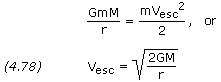which is independent of the mass of the spacecraft.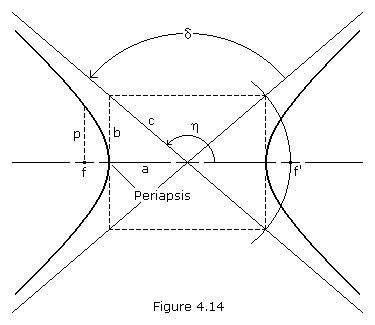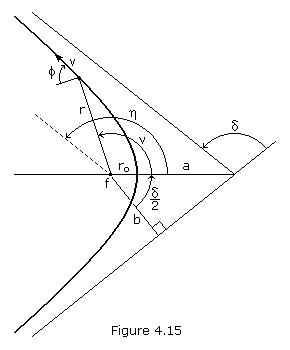A space vehicle that has exceeded the escape velocity of a planet will travel a hyperbolic path relative to the planet. The hyperbola is an unusual and interesting conic section because it has two branches. The arms of a hyperbola are asymptotic to two intersecting straight line (the asymptotes). If we consider the left-hand focus, f, as the prime focus (where the center of our gravitating body is located), then only the left branch of the hyperbola represents the possible orbit. If, instead, we assume a force of repulsion between our satellite and the body located at f (such as the force between two like-charged electric particles), then the right-hand branch represents the orbit. The parameters ab and c are labeled in Figure 4.14. We can see that c2 = a2+ b2 for the hyperbola. The eccentricity is,The angle between the asymptotes, which represents the angle through which the path of a space vehicle is turned by its encounter with a planet, is labeled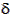. This turning angle is related to the geometry of the hyperbola as follows: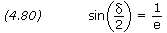If we letequal the angle between the periapsis vector and the departure asymptote, i.e. the true anomaly at infinity, we have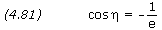If we know the radius, r, velocity, v, and flight path angle,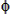, of a point on the orbit (see Figure 4.15), we can calculate the eccentricity and semi-major axis using equations (4.30) and (4.32) as previously presented. Note that the semi-major axis of a hyperbola is negative.

The true anomaly corresponding to known valves of rv andcan be calculated using equation (4.31), however special care must be taken to assure the angle is placed in the correct quadrant. It may be easier to first calculate e and a, and then calculate true anomaly using equation (4.43), rearranged as follows: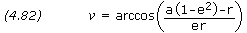Wheneveris positive,should be taken as positive; wheneveris negative,should be taken as negative.

The impact parameterb, is the distance of closest approach that would result between a spacecraft and planet if the spacecraft trajectory was undeflected by gravity. The impact parameter is,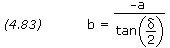Closet approach occurs at periapsis, where the radius distance, ro, is equal to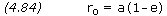p is a geometrical constant of the conic called the parameter or semi-latus rectum, and is equal toAt any known true anomaly, the magnitude of a spacecraft’s radius vector, its flight-path angle, and its velocity can be calculated using equations (4.43), (4.44) and (4.45).

Early we introduced the variable eccentric anomaly and its use in deriving the time of flight in an elliptical orbit. In a similar manner, the analytical derivation of the hyperbolic time of flight, using the hyperbolic eccentric anomalyF, can be derived as follows: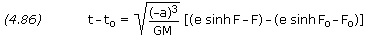where,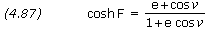Wheneveris positive, F should be taken as positive; wheneveris negative, F should be taken as negative.

Hyperbolic Excess Velocity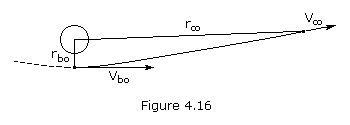If you give a space vehicle exactly escape velocity, it will just barely escape the gravitational field, which means that its velocity will be approaching zero as its distance from the force center approaches infinity. If, on the other hand, we give our vehicle more than escape velocity at a point near Earth, we would expect the velocity at a great distance from Earth to be approaching some finite constant value. This residual velocity the vehicle would have left over even at infinity is called hyperbolic excess velocity. We can calculate this velocity from the energy equation written for two points on the hyperbolic escape trajectory – a point near Earth called the burnout point and a point an infinite distance from Earth where the velocity will be the hyperbolic excess velocity, v. Solving for v we obtain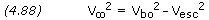Note that if v = 0 (as it is on a parabolic trajectory), the burnout velocity, vbo, becomes simply the escape velocity.

It is, or course, absurd to talk about a space vehicle “reaching infinity” and in this sense it is meaningless to talk about escaping a gravitational field completely. It is a fact, however, that once a space vehicle is a great distance from Earth, for all practical purposes it has escaped. In other words, it has already slowed down to very nearly its hyperbolic excess velocity. It is convenient to define a sphere around every gravitational body and say that when a probe crosses the edge of this sphere of influence it has escaped. Although it is difficult to get agreement on exactly where the sphere of influence should be drawn, the concept is convenient and is widely used, especially in lunar and interplanetary trajectories. For most purposes, the radius of the sphere of influence for a planet can be calculated as follows:where Dsp is the distance between the Sun and the planet, Mp is the mass of the planet, and Ms is the mass of the Sun. Equation (4.89) is also valid for calculating a moon’s sphere of influence, where the moon is substituted for the planet and the planet for the Sun.